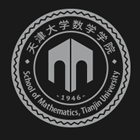﻿An Introduction to Euclidean Distance Matrix Optimization-天津大学数学学院

# An Introduction to Euclidean Distance Matrix Optimization

2019-09-02 09:55

Euclidean Distance Matrix (EDM) Optimization appears in a wide range of applications such as sensor network localization, protein conformation and multi-dimensional scaling (MDS). In particular, the classical MDS is equivalent to principle component analysis (PCA). This fundamental relationship between MDS and PCA makes the EDM optimization as one of the leading dimensionality reduction methods. This talks aims to provide a short introduction about EDM optimization, answering questions such as what consists of EDM optimization, what are the challenging issues and how to efficiently solve this class of matrix problems. We will use sensor network localization to illustrate the theory and state-of-the-art algorithms for EDM optimization.### 联系我们